Project
Project ref
Calcs for
Date

## Beam details

 Beam 63 x 170 mm C24 Grade Timber Timber strength class C24 Service class of timber 2 Width b = 63 mm Depth h = 170 mm## Span details

 Beam clear span Lcl = 1 m Bearing length Lb = 100 mm Beam effective span Leff = Lcl + (2 × (Lb / 2)) = 1.1 mDiagrams not to scale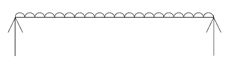Load 1: UDL - Sloping roof, 0° to 30°
 Dead load Fd,1 = 1.15 kN/m² × 2 m = 2.3 kN/m Imposed load Fi,1 = 0.75 kN/m² × 2 m = 1.5 kN/m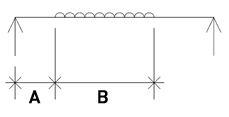Load 2: Partial UDL - Lightweight timber stud partitions, on floor plan
 Dimension to start of load, A A = 0.2 m Length of load, B B = 0.5 m Dead load Fd,2 = 1.15 kN/m² × 3 m = 3.45 kN/m Imposed load Fi,2 = 0.25 kN/m² × 3 m = 0.75 kN/m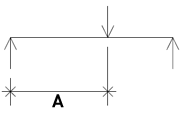## Reactions (unfactored)

 Dead Imposed Total Left reaction 2.68 kN 1.37 kN 4.06 kN Right reaction 3.62 kN 2.15 kN 5.77 kN

## Modification factors

 Timber service class modification factor K2 as table 16 Bending parallel to grain K2,ben = 1.00 Compression perpendicular to grain K2,per = 1.00 Shear parallel to grain K2,shr = 1.00 Mean & min modulus of elasticity K2,mod = 1.00 Load duration factor K3 = 1.25 Bearing modification factor K4 = 1.00 Depth factor (BS5268-2 clause 2.10.6) K7 = (300 / h)0.11 = 1.06 Load sharing modification factor (BS5268-2 clause 2.10.11) K8 = 1.00 Modulus of elasticity modification factor (BS5268-2 clause 2.9) K9 = 1.00

## Modulus of elasticity

 Timber minimum modulus of elasticity Emin = 7,200 N/mm² The minimum modulus of elasticity modified by the factor K9 should be used for deflections E = Emin × K2,mod × K9 = 7,200 N/mm²

## Section properties

 Area of section Area = b × h = 10,700 mm² Inertia of timber about xx axis Ixx = b × h³ / 12 = 25,800,000 mm4 Z to top edge of timber Z = b × h² / 6 = 303,000 mm³ Average density for C24 grade timber (BS 5268-2:2002 Table 8) ρmean = 420 kg/m³ Self weight (g = 9.81 m/s²) Fself = b × h × Leff × ρmean × g = 48.5 N

## Section design parameters

 Design bending moment Mb = 1,490,000 Nmm Design shear force Fve = 5,770 N

## Check bending stress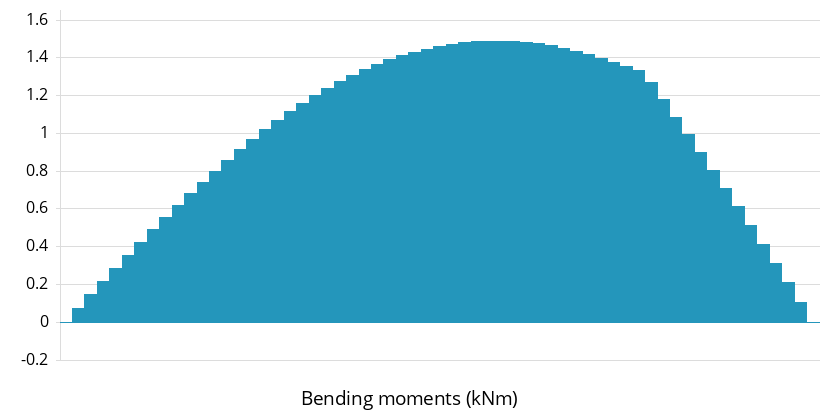Timber grade bending stress parallel to grain (BS5268-2 Table 8) σt,m,g,par = 7.5 N/mm² Permissible timber bending stress (factored) σt,m,adm = σt,m,g,par × K2,ben × K3 × K7 × K8 = 9.98 N/mm² Maximum bending moment M = 1.49 kNm Applied bending stress in timber σt,m,max = M / Z = 4.91 N/mm² Pass    σt,m,max <= σt,m,adm ( 4.905 N/mm² <= 9.979 N/mm² ) applied bending stress in timber within permissible

## Check deflection (including shear deflection as required by clause 2.10.7)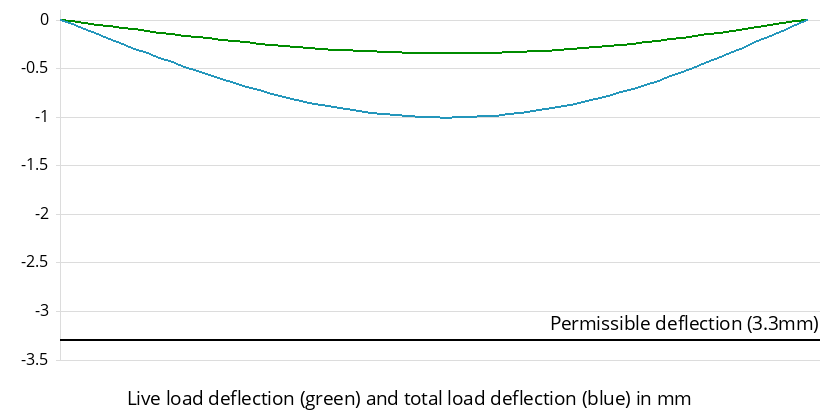## Check shear stress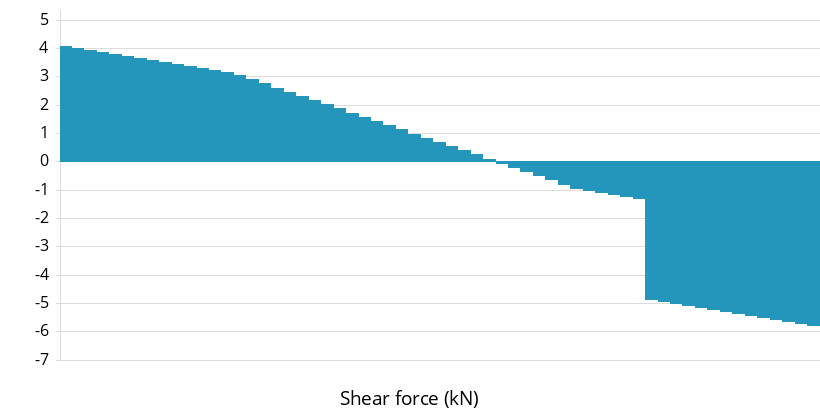No notches to occur at the critical shear position. Timber grade shear stress parallel to grain (BS5268-2 Table 8) τt,g,par = 0.71 N/mm² Permissible shear parallel to grain (factored) τt,adm = τt,g,par × K2,shr × K3 × K8 = 0.888 N/mm² Permissible shear force on timber Ft,adm = 2 × τt,adm × b × h / 3 = 6,340 N Design shear force Fve = 5,770 N Pass    Fve <= Ft,adm ( 5773 N <= 6337 N ) shear capacity of timber is greater than applied shear force, therefore OK

## Check bearing stress

 Timber grade compressive stress perpendicular to grain (BS5268-2 Table 8) σt,c,g,⊥ = 1.9 N/mm² Permissible compressive stress perpendicular to grain (factored) σt,c,adm = σt,c,g,⊥ × K2,per × K3 × K4 × K8 = 2.38 N/mm² Timber bearing stress on support σt,c,max = Fve / (Lb × b) = 0.916 N/mm² Pass    σt,c,max <= σt,c,adm ( 0.916 N/mm² <= 2.375 N/mm² ) bearing stress is less than permissible timber stress, therefore OK

## Design summary

 Permissible Applied/Actual Utilisation Result Shear force (kN) 6.34 5.77 91.1 % OK Bending stress (N/mm²) 9.98 4.91 49.2 % OK Bearing stress (N/mm²) 2.38 0.92 38.6 % OK Deflection (mm) 3.3 1.38 41.8 % OK

## Notes

This design is in accordance with BS 5268-2:2002 Structural use of timber - Part 2: Code of practice for permissible stress design, materials and workmanship.

The depth to width ratio of the timber does not exceed 5 and as per the requirements of BS 5268-2 Table 19 there is no risk of buckling under design load provided; The ends are held in position and compression edge held in line, as by direct connection of sheathing, deck or joists.

Timber to be covered, this calculation is not to be used for timber which is fully exposed to the elements.

Wane as allowed in BS 4978:2007 + A2:2017 is permitted.

DEMO